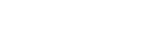# MATH106 - Finite Mathematics

## General

MATH

106

00209187

Lecture

### Description

Functions (linear, quadratic, exponential, and logarithmic), simple and compound interest, annuities,linear programming, and probability. Mathematical modeling and applications Prereq.: MATH 072 or high school advanced algebra with a satisfactory math placement score.

### Notes

Go to www.stcloudstate.edu/mathplacement Placement req prior to Registration

No Requisites

3

3

No

Yes

04

No

## Learning Outcomes

### Outcome

Algebraically solve linear, quadratic, rational, radical, exponential, and logarithmic equations and apply solutions to problems from business and economics.

### Outcome

Demonstrate an understanding of the concept of a function, including its domain and range; find the equations of, sketch graphs for, and perform transformations on, linear, quadratic, exponential and logarithmic functions.

### Outcome

Formulate and solve real-world application problems including the calculation of future and present values for simple annuities and interest-bearing accounts.

### Outcome

Graph linear inequalities and use linear programming to maximize or minimize linear objective functions subject to linear constraints.

### Outcome

Calculate the size of sets using combinations, permutations, the addition principle and the multiplication principle, and illustrate the results in tables or Venn diagrams.

### Outcome

Calculate the theoretical and empirical probability of an event occurring, including conditional probabilities and expected values.

### Outcome

Write logical, step-by-step solutions to problems and communicate solutions orally.

-

## Dependencies

No dependencies720 4th Avenue South
St. Cloud, MN 56301-4498
(320) 308-0121St. Cloud State University
A member of Minnesota State and committed to legal affirmative action, equal opportunity, access and diversity of its campus community (Full Statement).
© 2021 St. Cloud State University